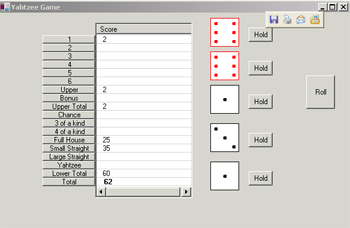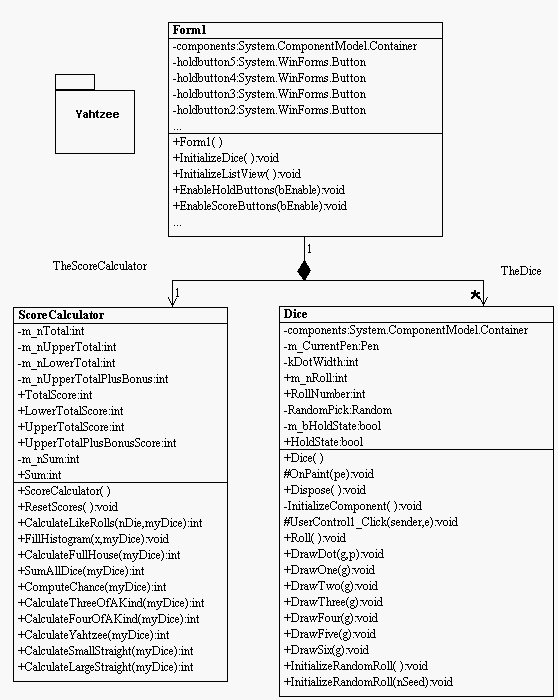Post

# Yahtzee Program using C#

• 48.1k
• 0
• 1The Visual C# environment has given the programmer the ability to create applications in RAD mode without the steep learning curve for the C++ programmer. (I do miss templates though!). The above application, yahtzee, is based on a popular poker-like dice game. The rules are below:

Rules

You have 3 rolls to get a desired score. You can only fill in each row once. On each roll you can 'hold' the dice you don't need to roll again. The idea is to fill in all the rows with the highest score you can. The first six rows are 1's, 2's,3's,4's,5's,6's respectively. If you decide to go for the 1's column, you add up all the ones showing at the end of your 3 rolls. For the 2's column add up all the 2's, etc. At the end of the game if your 1's, 2's, 3's, 4's, 5's, and 6's total 63 or greater, you get an additional bonus of 35 points. The lower half of the board has a few poker like names (and is similar to poker with 2 draws). If you have 3 of a kind of any number ( 3 of the same number) showing on the die faces, you can score this row, and the computer will give you the sum on all the faces of the dice. For example if you have 6,6,6,4,3, the computer will give you a score of 25. However, if you try to put a score this row and don't have at least 3 of the same, you get 0 in this row. If you have 4 of a kind of any number ( 4 of the same number) showing on the die faces, you can score this row, and the computer will give you the sum on all the faces of the dice. A full house is if you have 3 of a kind and 2 of a kind ( a pair). For example, 4,4,5,5,5 would be a full house with a pair of 4's and three 5's. A full house gives you a score of 25 points. A small straight is when you have 4 dice in consecutive order. For example 1,2,3,4 or 2,3,4,5 or 3,4,5,6 are all small straights. Small straights are worth 35 points. A large straight is when you have all 5 dice in consecutive order (e.g. 2,3,4,5,6) and this is worth 40 points. Yahtzee, (also coincidentally the name of the game) is when all 5 dice have the same value e.g. 1,1,1,1,1 or 5,5,5,5,5 are yahtzees. A yahtzee is worth a whopping 50 points!

Design

The design of yahtzee pretty much consists of three classes. The main form, Form1, which displays the board the dice and all the game buttons, the Dice User Control, which exhibits all the functionality of a 6-sided die, and the ScoreCalculator used to calculate the score of all the rows based on 5 dice and the particular yahtzee column.The yahtzee design diagram was reverse engineered into a UML diagram using WithClass 2000

The design exhibits a few powerful features of the C# environment. ListViews, User Controls, graphics and properties. In this first article we will talk about the dice control.

The Dice Control is a user control. It keeps track of the roll number and generates a random number for each roll. The dice control draws the die based on the dimensions of the client rectangle that the control is in. Each dice pattern can build upon other dice patterns. Below is how a six is drawn:

public void DrawSix (Graphics g)
{
DrawFour(g);
Rectangle rect =
this.ClientRectangle;
DrawDot(g,
new Point((rect.Left + kDotWidth),
(rect.Top+rect.Bottom - kDotWidth)/2));
DrawDot(g,
new Point((rect.Right - kDotWidth*2),
(rect.Top+rect.Bottom - kDotWidth)/2));
}

OnPaint is overriden in the control to paint the graphics for the dice based on the roll number:

protected override void OnPaint( PaintEventArgs pe )
{
// set up pens and brushes for the dice
Graphics g = pe.Graphics;
Pen blackPen =
new Pen( Color.Black, 1 );
Pen redPen =
new Pen( Color.Red, 1 );
Rectangle rect =
this.ClientRectangle;
SolidBrush whiteBrush =
new SolidBrush(Color.White);
g.FillRectangle(whiteBrush, rect);
// Check to see if the die is being held. If it is, paint it red
if (HoldState)
{
g.DrawRectangle(redPen, rect);
m_CurrentPen = redPen;
}
else
{
g.DrawRectangle(blackPen, rect);
m_CurrentPen = blackPen;
}
switch (m_nRoll)
{
case 1:
DrawOne(g);
break;
case 2:
DrawTwo(g);
break;
case 3:
DrawThree(g);
break;
case 4:
DrawFour(g);
break;
case 5:
DrawFive(g);
break;
case 6:
DrawSix(g);
break;
default:
break;
}
// toss the pens and brushes
blackPen.Dispose();
redPen.Dispose();
whiteBrush.Dispose();
}

Random numbers are generated for the dice using the Random class. The random class allows you to generate random numbers using a seed. Once you initialize the random instance with a seed, each # you ask for after will produce another random #. In this example we use NextDouble to get a # between 0 and 1.0. Then we force the range between 1 and 6 by scaling and translating up.

public void Roll ()
{
m_nRoll = (
int)(RandomPick.NextDouble() * 6) + 1;
}

One of the tricky things about dealing with random # generators is finding an initial seed. If we picked the same seed for all the dice, we would get the same roll for every die (that means a yahtzee every time, which isn't half-bad). But to give the game a challenge, we need to force a random result for each die. One way to do this is to use the system clock to seed the random # generator. Below is the routine we use to seed the dice user controls:

public
void InitializeDice()
{
try
{
TheDice = dice1;
TheDice = dice2;
TheDice = dice3;
TheDice = dice4;
TheDice = dice5;
dice1.InitializeRandomRoll(25 * (
int)(DateTime.Now.Millisecond));
dice2.InitializeRandomRoll(58 * (
int)(DateTime.Now.Millisecond));
dice3.InitializeRandomRoll(3 * (
int)(DateTime.Now.Millisecond));
dice4.InitializeRandomRoll(102 * (
int)(DateTime.Now.Millisecond));
dice5.InitializeRandomRoll(17 * (
int)(DateTime.Now.Millisecond ));
}
catch(Exception er)
{
System.Console.WriteLine(er.Message.ToString());
}
}

Unfortunately, each line is executed a little faster than a millisecond, so we put an additional random factor in by multiplying by some fixed value. Not the prettiest solution, but it seems to do the trick.

This is the first cut of yahtzee. For some, the game can get addictive, so keep in mind this is a programming example. In the future we'll update to play triple yahtzee so you can see some of those fancy subitem functions in ListViews. Enjoy!

Recommended Free Ebook
Similar Articles